# Computer Exercises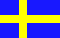This Appendix with Computer Exercises is also available in Swedish

## A 11.0 Introduction

The source of this appendix is my complete Fortran 90 tutorial in Swedish, Lärobok i Fortran 90/95.

These computer exercises are small variants of those used at the teaching of Fortran at Linköping University, both for the students of technology and for the students in the mathematics and science program.

When I write course directory below it is intended as an advice to the local teacher to make the required files available to the students. Of course, it is also possible to type them from the textbook, extract them from this HTML-file, or get them electronically from our course directory. Using UNIX it is easy to introduce symbolic names, so the course directory \$KURSBIB is /mailocal/lab/numt/TANA70/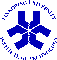Locally Fortran 77 (f77), Fortran 90 (f90), and Fortran 95 (f95), are all available on our workstations. Both the workstations and the file servers are from Sun. Previously we used Digital Equipment computers.

Further information on the course (including experiences from earlier students) is available (mainly in Swedish) on URL=http://www.mai.liu.se/~boein/kurser/TANA70/

The computers used for teaching at the Department of Mathematics have been replaced during 2001, from DEC machines to Sun machines. The new computers also mean a change in location of the course directory. You define the course directory by writing

```setenv KURSBIB /mailocal/lab/numt/TANA70/
```
A suitable environment is obtained with the TANA70setup command.

Further information on the Sun Fortran 90, and its system parameters, and hints on compilation are available.

Further information on the DEC Fortran 90, and its system parameters, hints on compilation, and some examples are still available.

## A 11.1 EXERCISE 1

### Runge-Kutta

The Pascal program below for solving an ordinary differential equation with the Runge-Kutta method is on the course directory, as the file \$KURSBIB/lab1_e.p. The assignment is to translate it from Pascal to Fortran. The output is required to include not only the numeric values but also suitable explanatory text. It is also valuable if the program can use other input values than those given in the Pascal code, 1 and 2, respectively.

The following material shall be handed to the teacher:

1. Listing of the translated program.
2. Listing (for example using the UNIX script) from a complete run of the four test cases
```        Number of steps      Step length

1               1
2               0.5
4               0.25
8               0.125
```
For those who do not know Pascal the recommended alternative is to get an elementary textbook on numerical methods and code Runge-Kutta directly in Fortran.

The task is to solve the differential equation y'(x) = x2 + sin(xy) with the Runge-Kutta method, using the initial value y(1) = 2 going to x = 2 and using the step sizes above. The formulas for the Runge-Kutta method are for example in the description of exercise 8.

```program RK1;
(* A simple program in Pascal for Runge-Kutta's method for a
first order differential equation.
dy/dx = x^2 + sin(xy)
y(1) = 2                                 *)
var     number, i : integer;
h, k1, k2, k3, k4, x, y : real;
function f(x,y : real) : real;
begin
f := x*x + sin(x*y)
end;
begin
number := 1;
while number > 0 do
begin
x := 1.0;
y := 2.0;
writeln(' Give the number of steps ');
if number >= 1 then
begin
writeln(' Give the step length ');
writeln('       x              y');
writeln(x, y);
for i := 1 to number do
begin
k1 := h*f(x,y);
k2 := h*f(x+0.5*h,y+0.5*k1);
k3 := h*f(x+0.5*h,y+0.5*k2);
k4 := h*f(x+h,y+k3);
x  := x + h;
y  := y + (k1+2*k2+2*k3+k4)/6;
writeln(x, y);
end;
end;
end;
end.
```
If you wish to run this program you have to replace the first line
```program RK1;
```
with the following extended line
```program RK1(INPUT, OUTPUT);
```
when running on a DEC UNIX system, but not on a Sun Solaris system.

## A 11.2 EXERCISE 2

### Horner's scheme and files

The program below is in Fortran 77 (or fix form Fortran 90) and is in the course directory as the file \$KURSBIB/lab2_e.f. The assignment is first to correct the errors in the program. There are no errors in the numerical algorithm (Horner's scheme) or in the comments in the program. But some programming errors are included in the presented program, several of these are based on a mixture of Fortran and Pascal.

When the program is believed to be correct (test with the equation x*x + 2 = 0), the second assignment is to modify the program so that it can accept data from either the keyboard or from a file \$KURSBIB/lab21.dat. The modifications are required to be done in such a way that the user can select if data are supposed to be input directly from the keyboard or from a file, in which case the user also will be required to give the name of the file.

Finally, check that the program also works for the file \$KURSBIB/lab22.dat.

Note that the program uses complex numbers, and that such values are input as pairs within parenthesis.

The program is also available in free mode Fortran 90 as \$KURSBIB/lab2_e.f90.

The following material shall be handed to the teacher:

1. Listing of the corrected and modified program.
2. Listing (at UNIX from script) of a run with the three test cases (x*x + 2 = 0), lab21.dat and lab22.dat.
The file lab2_e.f for computer exercise 2. Find the errors in the program!
```******************************************************************************
**      This program calculates all roots (real and/or complex) to an       **
**      N:th-degree polynomial with complex coefficients. (N <= 10)         **
**                                                                          **
**                 n        n-1                                             **
**       P(z) = a z  + a   z    + ... + a z + a                             **
**               n      n-1              1     0                            **
**                                                                          **
**      The execution terminates if                                         **
**                                                                          **
**                 1) Abs (Z1-Z0) < EPS       ==>   Root found = Z1         **
**                 2) ITER > MAX              ==>   Slow convergence        **
**                                                                          **
**      The program sets EPS to 1.0E-7 and MAX to 30                        **
**                                                                          **
**      The NEWTON-RAPHSON method is used:   z1 = z0 - P(z0)/P'(z0)         **
**      The values of P(z0) and P'(z0) are calculated with HORNER'S SCHEME. **
**                                                                          **
**      The array A(0:10) contains the complex coefficients of the          **
**      polynomial P(z).                                                    **
**      The array B(1:10) contains the complex coefficients of the          **
**      polynomial Q(z),                                                    **
**                  where P(Z) = (z-z0)*Q(z) + P(z0)                        **
**      The coefficients to Q(z) are calculated with HORNER'S SCHEME.       **
**                                                                          **
**      When the first root has been obtained with the NEWTON-RAPHSON 	    **
**      method, it is divided away (deflation).                             **
**      The quotient polynomial is Q(z).                                    **
**      The process is repeated, now using the coefficients of Q(z) as      **
**      input data.                                                         **
**      As a starting approximation the value STARTV = 1+i is used          **
**      in all cases.                                                       **
**      Z0 is the previous approximation to the root.                       **
**      Z1 is the latest approximation to the root.                         **
**      F0 = P(Z0)                                                          **
**      FPRIM0 = P'(Z0)                                                     **
******************************************************************************
COMPLEX      A(0:10), B(1:10), Z0, Z1, STARTV
INTEGER      N, I, ITER, MAX
REAL         EPS
DATA  EPS/1E-7/, MAX /30/, STARTV /(1,1)/
******************************************************************************
20    WRITE(6,*)  'Give the degree of the polynomial'
IF (N .GT. 10) THEN
WRITE(6,*) 'The polynomial degree is maximum 10'
GOTO 20
WRITE (6,*) 'Give the coefficients of the polynomial,',
, ' as complex constants'
WRITE (6,*) 'Highest degree coefficient first'
DO 30 I = N, 0, -1
WRITE (6,*) 'A(' , I, ') = '
30    CONTINUE
WRITE (5,*) '    The roots are','        Number of iterations'
******************************************************************************
40    IF (N GT 0) THEN
C     ******     Find the next root                                     ******
Z0 = (0,0)
ITER = 0
Z1 = STARTV
50        IF (ABS(Z1-Z0) .GE. EPS) THEN
C         ++++++ Continue the iteration until two estimates             ++++++
C         ++++++ are sufficiently close.                                ++++++
ITER = ITER + 1
IF (ITER .GT. MAX) THEN
C             ------ Too many iterations  ==> TERMINATE                 ------
WRITE (6,*) 'Too many iterations.'
WRITE (6,*) 'The latest approximation to the root is ',Z1
GOTO 200
ENDIF
Z0 = Z1
HORNER (N, A, B, Z0, F0, FPRIM0)
C             ++++++   NEWTON-RAPHSON'S METHOD                          ++++++
Z1 = Z0 - F0/FPRIM0
GOTO 50
ENDIF
C         ++++++++++++++++++++++++++++++++++++++++++++++++++++++++++++++++++++
100       WRITE (6,*) Z1, '         ',Iter
C         ****** The root is found. Divide it away and seek the next one *****
N = N - 1
FOR I = 0 TO N DO
A(I) = B(I+1)
GOTO 40
ENDIF
200   END

SUBROUTINE  HORNER(N, A, B, Z, F, FPRIM)
******    For the meaning of the parameters - see the main program      ******
******    BI and CI are temporary variable.                             ******

INTEGER N, I
COMPLEX A(1:10), B(0:10), Z, F, FPRIM, BI, CI

BI = A(N)
B(N) = BI
CI = BI

DO 60  I = N-1, 1, -1
BI = A(I) + Z*BI
CI = BI + Z*CI
B(I) = BI
C            ++++++ BI = B(I) for the calculation of P(Z)               ++++++
C            ++++++ CI  for the calculation of P'(Z)                    ++++++
60    CONTINUE

F = A(0) + Z*BI
FPRIM = CI

RETURN
END
******   END HORNER'S SCHEME                                            ******

******   This program is composed by Ulla Ouchterlony 1984              ******
******   The program is translated by Bo Einarsson 1995                 ******
```
Comments. I recommend the following process at the solution of this exercise.
1. Read the program and correct the errors you find.
2. Run the program to see if it works satisfactorily.
3. If it does not work properly, correct any new errors and return to item 2. If you did not find any new errors, recompile the program using a check of not declared variables. This can on our DEC system be done with
```                f77 -u lab2_e.f
a.out
```
Using a modern system, for example Fortran 90, you put the statement IMPLICIT NONE first in booth the main program and the subroutine. The command -u has another meaning on the Absoft compiler.
4. Correct the additional errors found now, and return to item 2. If you did not discover any new errors, recompile using checking of array indices. On some systems, as on our DEC system and with the NAG Fortran 90, this can be done with
```                f77 -C lab2_e.f
a.out
```
With the Absoft compiler you write f90 -Rb and with the Intel ifort 8.0 you write ifort -CB.
6. When the program seems to work properly, start to modify the program to handle also input data from a file.
7. The commands to open and close a file are given in Appendix 2.
8. At list-directed input of text the text characters should preferably be given within apostrophes (is required by some systems). This is not user-friendly! I therefore recommend formatted input in this case. If only one character is to be given, use FORMAT(A1), if thirteen characters are to be given, use FORMAT(A13).
9. Remember that each read or write statement starts with a new record (line). This is also the case within an explicit DO-loop. If several data values are on the same line, they have to be read with the same statement. This can be achieved by using the implicit DO-loop.

The explicit DO-loop below will write seven lines, each one with one value of X

```	DO I = 1, 13, 2
WRITE(*,*) X(I)
END DO
```

The implicit DO-loop below will write only one line, but with seven values of X

``` 	WRITE(*,*) ( X(I), I = 1, 13, 2)
```
10. Please note that since Newton-Raphson is only convergent close to the root there is no guarantee that the program will work for arbitrary input values.
11. According to the standard, complex numbers are to be written with a parenthesis around the real part and the imaginary part, even if the imaginary part is zero, as in (1.0, 0.0). Some compilers, for example the Intel ifort, permit that you in these cases only write the real part, as 1.0, the imaginary part will then automatically be assigned the value zero.

I recommend that you test if your system permits this simplified form for input, but please use it only for input via the keyboard and never at input from a file (of portability reasons).

## A 11.3 EXERCISE 3

### Factorial and Bessel

This is computer exercise number three, and it consists of writing two small programs in the programming language Fortran 77.

### Exercise 3 a)

Write a function in Fortran 77 with a main program for evaluation of the factorial function. Use only integers. Write the main program in such a way that it asks the user for an integer, for which the factorial is to be calculated. Make a test run and evaluate 10!

### Exercise 3 b)

Write a program in Fortran 77 for tabulating the Bessel function J0(x). Use for example the NAG Fortran 77 Library, or the NAG Fortran 90 Library, or a similar library, for obtaining values of this function. Write the main program in such a way that it asks for an interval for which the function values are to be evaluated.

Make a test run and print a table with x and J0(x) for x = 0.0, 0.1, ... 1.0. Make the output to look nice!

At the use of the NAG Fortran 77 library the NAG User Notes are very useful. They describe the system specific parts of the library.

The required Bessel function in the NAG library is S17AEF and has the arguments X and IFAIL and in this order, where X is the argument for the Bessel function in single or double precision, and where IFAIL is an error parameter (integer). The error parameter is given the value 1 before entry, and is checked at exit. If it is 0 at exit, everything is OK, if it is 1 at exit, the magnitude of the argument is too large.

If the NAG library is installed in the normal way it is linked with the command

```        f77 prog.f -lnag
```

It is also permitted to use the new NAG Fortran 90 library. Please note that the functions have different names in this library (our Bessel function is nag_bessel_j0), and is linked with

```        f90 prog.f90 -lnagfl90
```
The error handling is done differently in the NAG Fortran 90 library, there is no IFAIL parameter. For this exercise you may ignore the error handling if you use this library. If an error occurs, it will be automatically signalled.

If you use Fortran 90 for your own program but has the NAG library available only in Fortran 77 special care is necessary, see chapter 15, Method 2.

On Sun you compile and link with

```        f90 prog.f -lnag -lF77
```
and on DEC ULTRIX with
```        f90 prog.f -lnag -lfor -lutil -li -lots
```

On DEC UNIX -lfor -lutil -lots are available.

It is very important to note in which precision the NAG library is made available on your system. Using the wrong precision will usually give completely wrong results!Local information: The NAG library is not available on the Sun system in Linköping.

### Exercise 3 c)

Since the Mathematics Department in Linköping last year did not wish to pay for a license of the NAG library we have made an alternative exercise, which uses a mini library written by myself.

Write a program in Fortran 77 for tabulating the Bosse function Bo(x). Write the main program in such a way that it asks for an interval for which the function values are to be evaluated.

Make a test run and print a table with x and Bo(x) for x = 0.0, 0.1, ... 1.0. Make the output to look nice! Investigate also what happens with the arguments +800 and -900!

The Bosse function BO is in the library libbosse.a on the course directory and has the arguments X and IFAIL and in this order, where X is the argument for the Bosse function in single or double precision, and where IFAIL is an error parameter (integer). The error parameter is given the value 1 before entry, and is checked at exit. If it is 0 at exit, everything is OK, if it is 1 at exit, the argument is too large, if it is 2 at exit, the argument is too small. If the error parameter is zero on entry and an error occurs the program will stop with an error message from the library error handling subroutine, and not return to the user's program. The error handling is similar to the one in the NAG Fortran 77 library.

If the Bosse library is installed in the normal way it is linked with the command

```        f77 prog.f -L\$KURSBIB -lbosse
```

If you use Fortran 90 for your own program but you still have the Bosse library available only in Fortran 77 special care is necessary, see chapter 15, Method 2, see also the discussion above in exercise 3b.

To make life simpler we have however made also a Fortran 90 version of the Bosse library, which is used with

```        f90 prog.f90 -L\$KURSBIB -lbosse90
```
Remark 1. It is worthwhile to note that on some systems the order of the directory and the library has to be reversed, as in
```        f90 prog.f90 -lbosse90 -L\$KURSBIB
```

Remark 2. It is very important to note in which precision the Bosse library is made available on your system. Using the wrong precision will usually give completely wrong results!Local information: The Bosse library is in double precision on the Sun system.

## A 11.4 EXERCISE 4

### Factorial and Runge-Kutta

This is computer exercise number four, and means full transition from Fortran 77 to Fortran 90. Free form of the source code is required!

### Exercise 4 a)

Write a recursive function in Fortran 90 with a main program for evaluation of the factorial function. Use only integers. Write the main program in such a way that it asks the user for an integer, for which the factorial is to be calculated. Make a test run and evaluate 10!

Compare with Exercise 3 a.

### Exercise 4 b)

Write a program in Fortran 90 for the numerical solution of a system of ordinary differential equations using the classical Runge-Kutta method. The following system is given
```        y'(t) = z(t)                        y(0) = 1
z'(t) = 2*y*(1+y^2) + t*sin(z)      z(0) = 2
```
Calculate an approximation to y(0.2) using the step size 0.04. Repeat the calculation with the step sizes 0.02 and 0.01. The functions representing the differential functions are to be given as internal functions. It is therefore not permitted (in this exercise) to use the usual external functions.

Starting values of t, y and z are to be given interactively, as well as the step size h.

Note that the requirement not to use external functions makes it a little more difficult to use a subprogram for the Runge-Kutta steps (in this case also the subprogram has to be internal). The aim of this requirement is to give the student an opportunity to use the new internal functions of Fortran 90.

The formulas for Runge-Kutta at systems are available here as an dvi-file and here as an HTML-file (which requires indices)! November 29

## A 11.5 EXERCISE 5

### Linear systems of equations and files

This is computer exercise number five. It consists of writing in Fortran 90 one main program and a number of subprograms, all in double precision, for the solution of a common numerical problem. The problem is to solve a linear system of equations Ax = b, using an available routine for the numerical task. At least four hours are suggested for this exercise.

Remarks:

• It is recommended to have a menu in the main program, preferably using SELECT CASE, where the user selects which subprogram to execute. After execution of that subprogram control is returned to the main program for a new choice.

• The main program uses LASMAT, LASVEK, MATIN, and VEKIN to read the matrix A and the vector b, calls the solver LOS and writes (prints) the matrix A, the right hand side vector b and the solution vector x with the subprograms MATUT and VEKUT.

• The program is required to test for example that you do not try to write the solution with VEKUT before it has been evaluated.

• If the dimension of the matrix and the vector do not agree, then the subprogram LOS or the main program is required to give an error message. An error message is also required if the routine SOLVE_LINEAR_SYSTEM detects that the matrix is singular.

• The main program HUVUD utilizes LASMAT, LASVEK, MATIN, and VEKIN in order to get the matrix A and the vector b, calls the solver subprogram LOS and prints the matrix A , the right hand side b and the solution x with the subprograms MATUT and VEKUT.

• The dimension in all the programs shall use the dynamic possibilities of Fortran 90. One alternative is to use pointers at the specification, see chapter 12. Another and better possibility is to introduce SAVEd arrays in a MODULE. A simple example of this is available.

• The program is required to return to the starting point after each calculation, and ask for a new matrix and/or vector. It has to be possible to use a stored set of matrix and vector, without manual input of a lot of values.

• In the exercise it is included the essential task of performing any additional specifications, test the reasonableness of the given values, and to construct suitable test matrices and vectors.

The first subprogram LASMAT is used to interactively input the floating point values of a quadratic matrix. The subprogram is required to ask for the dimension and give the user the choice of providing from the keyboard either all the values of the matrix, or only those which are different from zero, The matrix shall then be stored in a file, using maximal accuracy and minimal storage space. The possible sparsity of the matrix is however not permitted to be exploited at this storage process. The subprogram shall ask for the name of the file, but the file type (extension) will be .mat. The user is not permitted to give the file type.

Remarks to LASMAT:

• It is recommended to have two modes for input, one for the conventional row by row input of a matrix, and another mode where row number, column number, and the corresponding value, are being inputted element for element in any order. The first mode is excellent for the input of the two test matrices below!

• The requirement maximal accuracy and minimal storage space implies the use of unformatted output.

The second subprogram LASVEK is used to interactively input the floating point values of a vector. The subprogram is required to ask for the dimension and give the user the choice of providing from the keyboard either all the values of the vector, or only those which are different from zero, The vector shall then be stored in a file, using maximal accuracy and minimal storage space. The possible sparsity of the vector is however not permitted to be exploited at this storage process. The subprogram shall ask for the name of the file, but the file type (extension) will be .vek. The user is not permitted to give the file type.

The third subprogram MATIN reads a matrix from the file with the given name and stores it as an array (matrix) in the subprogram, in such a way that the matrix is available also in the calling subprogram. The user is not permitted to give the file type.

The fourth subprogram VEKIN reads a vector from the file with the given name and stores it as an array (vector) in the subprogram in such a way that the vector is available also in the calling subprogram. The user is not permitted to give the file type.

The fifth subprogram MATUT prints a matrix on paper, with rows and columns in the normal way if the dimension is at most 10, and in an easily understandable way if the dimension is larger than 10. The available 132 positions of a typical line printer are to be utilized as well as possible. Output on paper is under UNIX not done directly, so you will have to first write to a file, which is then moved to paper with the UNIX print command lpr. Also using Microsoft Windows you create a text file which is then written by a Windows command.

The sixth subprogram VEKUT prints a vector on paper, preferably in the transposed form (line-wise). Please note that there are both a right hand side and a solution vector to print! It is therefore recommend to let this subprogram pass information on which vector is printed.

The seventh subprogram LOS solves the system of equations through a call to the provided routine SOLVE_LINEAR_SYSTEM, which is given in double precision. No changes are permitted in that routine, and it is not permitted to put it into a module!

A compulsory test example follows

```		 33	 16	 72			-359
A =	-24	-10	-57		b =	 281
-8	 -4	-17			  85
```

Another compulsory test example is the following (with a 4 x 4 matrix)

```                  2    3    0    0                        1
0    0   1.8  10.1                     11
A =                                     b =
0.2  4.3   5    0                       42
0   0.8   7    7                      109
```

The students are required to hand in a complete program listing, and results from actual runs with matrices of the order 3, 4, 10, and 12, including input of a sparse matrix.

• Suggestion 1. Test also on the trivial case with dimension equals 1 for both the matrix and the vector.

• Suggestion 2. The provided routine may not be changed. The user therefore has to use an INTERFACE.

• Suggestion 3. I recommend you to perform the first compilation one routine (program unit) at a time, in order not to be flooded with error messages. When all the program units compile four different ways of combining them into a program exist.

I assume that the main program is on the file huvud.f90, the subroutine LASMAT on the file lasmat.f90, and so on, and that a possible complete program is on the file labb5.f90.

1. Compile all the routines separately but at the same time and with the same command,
```     f90 huvud.f90 lasmat.f90 lasvek.f90 ... los.f90 solve.f90
```
Execute with
```     a.out
```
2. Compile all the routines separately and use the object modules for linking,
```     f90 -c huvud.f90
f90 -c lasmat.f90
f90 -c lasvek.f90
...
f90 -c los.f90
f90 -c solve.f90
f90 huvud.o lasmat.o lasvek.o ... los.o solve.o
```
Execute with
```      a.out
```
When one routine has been changed, now only that one has to be recompiled, followed by linking of all the routines. This method is much faster!

3. Combine all the files into one large and compile,
```      f90 labb5.f90
```
Execute with
```      a.out
```
This method is very slow!

4. Compile all the routines separately but using the make command. Execute with labb5. This is advanced UNIX! A suitable makefile is below and in the course directory. This is the best method!
```labb5:  huvud.o lasmat.o lasvek.o matin.o vekin.o \
matut.o vekut.o los.o solve.o
f90 -o labb5 huvud.o lasmat.o lasvek.o matin.o vekin.o \
matut.o vekut.o los.o solve.o
huvud.o: huvud.f90
f90 -c huvud.f90
lasmat.o: lasmat.f90
f90 -c lasmat.f90
lasvek.o: lasvek.f90
f90 -c lasvek.f90
matin.o: matin.f90
f90 -c matin.f90
vekin.o: vekin.f90
f90 -c vekin.f90
matut.o: matut.f90
f90 -c matut.f90
vekut.o: vekut.f90
f90 -c vekut.f90
los.o:  los.f90
f90 -c los.f90
solve.o: solve.f90
f90 -c solve.f90
```
The above is used by moving the file makefile to your directory and when you wish to compile you write only make in the terminal window. Then only those files that have been changed as compared with the previous make command are recompiled (or all files if it is the first time), then all the object modules are linked to a program, ready to run with the command labb5.

If you have used other file names you have to make the appropriate modifications to the file makefile.

In principle make works in such a way that if an item which is after a colon : has a later time than that before the colon, the command on the next line is performed.

Remark. The backslash \ indicates a continuation line in UNIX.

The routine SOLVE_LINEAR_SYSTEM is available in single precision in the course directory with the name solve1.f90 and in double precision with the name solve.f90, and looks as follows. The single precision version is discussed at length in the Solution to Exercise (11.1).

```SUBROUTINE SOLVE_LINEAR_SYSTEM(A, X, B, ERROR)
IMPLICIT NONE
! Array specifications
DOUBLE PRECISION, DIMENSION (:, :), INTENT (IN)  :: A
DOUBLE PRECISION, DIMENSION (:),    INTENT (OUT) :: X
DOUBLE PRECISION, DIMENSION (:),    INTENT (IN)  :: B
LOGICAL, INTENT (OUT)                            :: ERROR

! The work area M is A extended with B
DOUBLE PRECISION, DIMENSION (SIZE (B), SIZE (B) + 1) :: M
INTEGER, DIMENSION (1)                               :: MAX_LOC
DOUBLE PRECISION, DIMENSION (SIZE (B) + 1)           :: TEMP_ROW
INTEGER                                              :: N, K

! Initiate M
N = SIZE (B)
M (1:N, 1:N) = A
M (1:N, N+1) = B

! Triangularization phase
ERROR = .FALSE.
TRIANG_LOOP: DO K = 1, N - 1
! Pivotering
MAX_LOC = MAXLOC (ABS (M (K:N, K)))
IF ( MAX_LOC(1) /= 1 ) THEN
TEMP_ROW (K:N+1 ) = M (K, K:N+1)
M (K, K:N+1) = M (K-1+MAX_LOC(1), K:N+1)
M (K-1+MAX_LOC(1), K:N+1) = TEMP_ROW (K:N+1)
END IF

IF (M (K, K) == 0.0D0) THEN
ERROR  = .TRUE.     ! Singular matrix A
EXIT TRIANG_LOOP
ELSE
TEMP_ROW (K+1:N) = M (K+1:N, K) / M (K, K)
M (K+1:N, K+1:N+1) = M (K+1:N, K+1:N+1)  &
- SPREAD( TEMP_ROW (K+1:N), 2, N-K+1) &
* SPREAD( M (K, K+1:N+1), 1, N-K)
M (K+1:N, K) = 0 ! These values are not used
END IF
END DO TRIANG_LOOP

IF (M (N, N) == 0.0D0) ERROR  = .TRUE.  ! Singular matrix A

! Back substitution
IF (ERROR) THEN
X = 0.0D0
ELSE
DO K = N, 1, -1
X (K) = M (K, N+1) - SUM (M (K, K+1:N) * X (K+1:N))
X (K) = X (K) / M (K, K)
END DO
END IF

END SUBROUTINE SOLVE_LINEAR_SYSTEM
```

NB: The routine above uses summation of empty vectors, that is in principle SUM(vector(N+1:N)), where the vector is dimensioned to have at most N elements, that is, we have an index out of bounds. The rule for the summation routine SUM is such that in this case (sum zero elements) it will give a result zero. Compare with the DO-loop backwards. If you compile with index check, for example with f90 -C solve.f90, you may obtain an error message. I have therefore modified the routine to avoid this possibility, the modified versions are in the library (course directory).

#### Exercise 5, Alternative a)

This is the Exercise 5 as described above, but an additional requirement is to use pointers at the specification of the array to store the matrix, using the method in chapter 12.

#### Exercise 5, Alternative b)

This is the Exercise 5 as described above, but an additional requirement is to use a module and an allocatable and saved array at the specification of the array to store the matrix, using the alternative method in chapter 12 and which is illustrated in an example. Pointers are not permitted.

#### Exercise 5, Alternative c)

This is the Exercise 5 as described above, but instead of using the given routine solve.f90 for the solution of the system of equations, you have to select and use a suitable routine from the Fortran 77 NAG library. Complete documentation is available in printed documents from NAG and via the NAG Web-site NAG Fortran Library.

```        f90 prog.f90 -lnag
```

When the NAG library is compiled with Fortran 77, it may be necessary to use method 2 from chapter 15, that is you compile and link the necessary libraries with an implementation dependent command.

It is also helpful to use the command naghelp when available.

At the the specification of the array to store the matrix, you may chose either the method in a) or b) above, or perhaps invent a third method.

If the makefile is used, the reference to solve.f90 must be changed.

#### Exercise 5, Alternative d)

This is the Exercise 5 as described above, but instead of using the given routine solve.f90 for the solution of the system of equations, you have to select and use a suitable routine from the Fortran 90 NAG library. Complete documentation is available in printed documents from NAG and via the NAG Web-site NAG fl90 and FL90plus Libraries.

```        f90 prog.f90 -lnagfl90
```

At the the specification of the array to store the matrix, you may chose either the method in a) or b) above, or perhaps invent a third method.

If the makefile is used, the reference to solve.f90 must be changed.

#### Exercise 5, Alternative e)

This is the Exercise 5b as described above, but the former requirement not to use the sparsity of the matrix at storage is replaced by a requirement to use it, but only in the subprograms LASMAT and MATIN. This can be done as in Appendix 3, section 12, with the new user-defined data type NONZERO. The file (matrix) is now to be stored with the extension .spr, still using maximal accuracy and minimal storage.

## A 11.6 EXERCISE 6

### Factorial

This is computer exercise number six, and it consists of writing a few variants of a program to evaluate the factorial.

Write a function in Fortran with an accompanying man program for evaluating the factorial function. It is recommended to write the main program in such a way that it requests the values of the integers for which the factorial is to be evaluated. Make test runs with different integers as input.

1. Start with integers as the data type in the function. Note the largest integer which gives a valid result!
2. Switch to real numbers REAL in the function. The input value should remain an integer. Note the largest integer which works! There are now two possible answers, one for the largest value which gives a completely accurate result, another one for the largest value which gives an approximatively correct value. You may also have to distinguish between correct value in binary form and correct value in the decimal representation!
3. Repeat with real numbers in DOUBLE PRECISION in the function.
4. Repeat with real numbers in quad precision, if available.

## A 11.7 EXERCISE 7

### Bessel function

This is computer exercise number seven, and it consists of writing a program to evaluate the Bessel function J0(z).

Bessel functions are solutions to the Bessel differential equation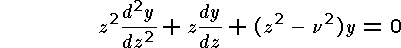named for Friedrich Wilhelm Bessel (1784 - 1846), who was the first to study its solutions as part of his research in celestial mechanics. The constant v indicates the "order" of the equation. It is possible to show that in the case v = 0 the seriesis one of the linearly independent solutions, and it is usually denoted J0(z).

The series is convergent in the whole complex plane, but due to possible cancellation you are only requested to consider the disc | z | <= 1.

You are supposed to write a function in Fortran using single precision which evaluates J0(z) and warns the user if the argument is too large (in its absolute value), but without using any extra input or output arguments.

It is required that the function is written as generic, which means one version for complex arguments and one for real arguments, the relevant version is chosen automatically. A suitable termination criteria at the summation is when the newly added term does not contribute to the sum. The function must reside in a module, which is used by the main program described below.

Write a main program which uses the function to generate a table of J0(z) on the three lines Re(z) = -0,5; Re(z) = +0,5 and Im(z) = 0 with a suitable step size.

Computer exercise 7 b is to modify the program to handle not only single precision, but also double and quad precision. The program is to consist of modules and a main program, no other program units.

## A 11.8 EXERCISE 8

### Runge-Kutta

This is computer exercise number eight, and it consists of writing a program in Fortran for solving a system of ordinary differential equations with the classical Runge-Kutta method. For the system below, where a line above a character denotes that it is a vector,the Runge-Kutta method is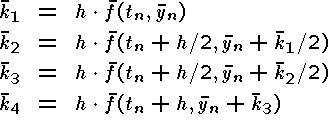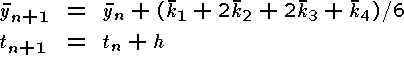You are supposed to write a subroutine with the input parameters tn, yn, h, and f, and with the output parameters tn+1 and yn+1. Here y is a vector and f(t, y) is a vector-valued function with the scalar argument t and the vector argument y.

Apply your program on at least two of the problems below. Compute approximations to y(0.2) with using the step lengths h = 0.04; 0.02 and 0.01. Finally, a manual Richardson extrapolation is recommended.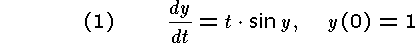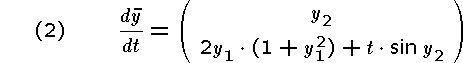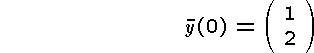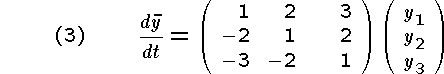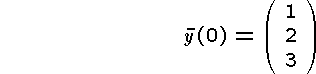Program and the results of the execution are to be presented.

Remarks:

• It is recommended to let the program consist of a main program, three functions (for the three right hand sides in the differential equations) and a subroutine (for the Runge-Kutta calculation). I recommend that the three functions are put into a module, and the subroutine into another module.

• In the subroutine for Runge-Kutta a formal argument is used for the function, which we may call F(T,Y). The problem here is that the dimension of Y may be one, two, or three. An INTERFACE is therefore required, for example
```             INTERFACE
FUNCTION F(T,Y) RESULT (FOUT)
REAL                     :: T
REAL, DIMENSION(:)       :: Y
REAL, DIMENSION(SIZE(Y)) :: FOUT
END FUNCTION F
END INTERFACE
```
The program will at execution recognize the present dimension of Y and assign that same dimension to the function F (and the result variable FOUT). The three actual functions have to be written in a consistent way, even the first one has to be written as a vector (with unknown length, which will evaluate to one), and not as a scalar.

• If you do not use modules two more INTERFACEs are required!

## A 11.9 EXERCISE 9

### User-defined data type

#### Part 1.

Write a program which defines two user-defined data types. The first is to contain relevant personal information, such as Christian name, middle initial, surname, sex, age, profession, and possibly some other information which you find suitable. The second contains an address in a suitable form. Store your own data into these data types and let the program write your data approximately as
```My name is Bo G. Einarsson. I am a 67 years old male
politician, and I live on Ekholmsvägen 249 in Linköping.
```

#### Part 2.

Use these two data types to create a new data type "family", which contains the names of the father, mother and two children, and their common address. Create test data (preferably fictious ones) and let the program write a family summary. Note especially the problems with syllabication and new lines.

Remark:

User-defined data types are discussed in Chapter 12, section 3 and Appendix 3, section 12.

You may use a static length of all the character strings. Character strings with variable length are in an extension to the Fortran standard. At the output unnecessary spaces are not permitted. The function LEN_TRIM gives the length of a character string without its trailing blanks.

You may further assume that the used name is the first of the Christian names and there is only one extra name, which initial should be used.

## A 11.10 EXERCISE 10

Write your own program as a function to evaluate the sine function for small values of the magnitude of the argument X, that is for -pi/2 < x < pi/2. Use the Maclaurin expansion. Desired accuracy should be given as an optional second argument as in MY_SIN(X, ACC=Y). The precision may be single, double, or quad. Use the generic facility!

If the second and optional argument is missing a default accuracy shall be used!

Also write a test program and compare with the intrinsic SIN(X). The precision may be single, double, or quad.

Expand the program to arbitrary real values of X, but no longer requiring full accuracy.

## A 11.11 EXERCISE 11

### Unstable algorithm

This exercise analyzes an unstable algorithm. Write a program to evaluate the integrals I0, I1, ... ,I20, where
```   In = Integral from 0 to 1 of x^n exp(-x) dx
```
using the recursion formula
```   I0 = 1 - 1/e

In = -1/e + n In-1      (n = 1, 2, 3, ...)
```
Write the program in such a way that it uses either single or double precision, depending on a certain module defining the precision.

Look at the results and compare with simple analytical estimates!

There is a trick to evaluate these integrals correctly! Do you remember the trick? If you start with an initial value with six significant digits you may end up with a result which is pure rubbish, but if you start with a value with no significant digits at all you may end up with six significant digits in the result! Write also this program!

## A 11.12 EXERCISE 12

### Interval arithmetics

The compiler from Sun has an intrinsic system for interval arithmetics. An example on the usage of the Newton-Raphson method is in the course directory in the file ia_newton.f90.

Your assignment is to modify this program to determine all zeroes of the function

f(x) = exp(-10x2) + cos(pi*x)

in the interval [-10; 10]. Use thereafter the method independent error estimate to verify the two roots that are smallest by magnitude.

Compile with the command

```f95 -xia file.f90
```
in order to activate the Sun interval arithmetics extensions. Further information is available on the Sun page.

I recommend that the accuracy parameters f_eps and root_eps are diminished from the present value 10-5 to be not greater than 10-10.

## A 11.13 EXERCISE 13

### Binary stars

The brightness of a binary star varies as follows. At time t = 0 its magnitude is 2.5, and it stays at this level until t = 0.9 days. Its magnitude is then determined by the formula
``` 3.355 - ln(1.352 + cos(pi(t-0.9)/0.7))
```
until t = 2.3 days. Its magnitude is then 2.5 until t = 4.4 days, and is then determined by the formula
``` 3.598 - ln(1.998 + cos(pi(t-4.4)/0.4))
```
until t = 5.2 days. Its magnitude is then 2.5 until t = 6.4 days, after which the cycle repeats with a period of 6.4 days.

Write a function which will input the value of the time t and output the brightness of the star at that time. Write a main program to print a graph of the brightness as a function of time in the interval t = 0 to t = 25.

If you do not have access to a Fortran plotting package you may use another package to plot, for example MATLAB.

## A 11.14 EXERCISE 14

### Logical function of character arguments

Write a logical function which has two CHARACTER arguments, and which returns the value .TRUE. if the first argument contains the second, and .FALSE. otherwise. Thus, if the function is called WITHIN, then
```   WITHIN("Just testing", "test")
```
will return .TRUE., while
```   WITHIN("Just testing", "Test")
```
will return .FALSE.

Use one or more of the many intrinsic functions!

Test your function with a main program which inputs pairs of CHARACTER strings from the keyboard and uses the result of a function reference to cause one of the following forms of message to be displayed

```   The phrase 'test' is contained within "Just testing"

The phrase 'Test' is not contained within "Just testing"
```

## A 11.15 EXERCISE 15

### Dot and vector products

Write two generic functions for the dot product and the vector product of two three-dimensional vectors. As arguments floating point numbers in single, double, or quad, precision are permitted, but for simplicity only one type at each call.

Use these two to write a third function to calculate the scalar triple product, defined as

``` [abc] = a . (b x c)
```

Finally write a main program which reads the three vectors a, b and c and writes the dot or scala product a . b and the vector product b x c and also the scalar triple product [abc].

Test on the following vectors:

```a = (  1 10  20 )T

b = (  5  8   9 )T

c = ( 12 36 108 )T
```
and
```a = (  1 sqrt(2)  sqrt(3) )T

b = (  5    8       9  )T

c = (  2    3       8 )T
```
where sqrt means the square root (in the required precision).

Note. The vector product is defined by

``` c = a x b

c1 = a2 b3 - a3 b2
c2 = a3 b1 - a1 b3
c3 = a1 b2 - a2 b1
```

## A 11.16 Exercise 16

### Ecological differential equation

In this exercise we consider a system of the second order of differential equations of the first order. The system is similar to a common ecological problem with rabbits, prey, and foxes, predator.
```
u'(t) =  u(t)·[A - 0.1·v(t)]  + 0.015·t,     u(0) = alpha

v'(t) =  v(t)·[0.02·u(t) - 1] + 0.0075·t,    v(0) = beta

```

All entities are normalised, therefore the real time scale is not known, we can call the time unit "year". We indicate the number of rabbits with u(t) and the number of foxes with v(t). It is a good idea to consider the numbers as thousands!

The solution to this system of differential equations is to be programmed in Fortran, using the Runge-Kutta method and double precision. In addition to calculating the number of rabbits and foxes the program is also supposed to evaluate the error in the number of rabbits and foxes, respectively, depending on that the parameter A is not known exactly as 1 but instead only as 1 + dellta. The computations are to be performed using the values A = 1 and dellta = 0.02. The formula yo be used is

```
DELTAun+1 = [1 + h·(1 - 0.1·vn)]·DELTAun - 0.1·h·un·DELTAvn + h·un·dellta

DELTAvn+1 = [1 + h·(0.02·un - 1)]·DELTAvn + 0.02·h·vn·DELTAun
and
DELTAu0 = 0
DELTAv0 = 0
dellta = 0.02

```

It is actually using the Euler method, but you may use it for the error calculation in order to simplify the programming.

Remark: The error is called dellta because delta is a reserved name for fix point arithmetic in Ada (the original language of this exercise).

Your assignment is to run the program with two different sets of the initial values alpha (thousands of rabbits at the starting time) and beta (thousands of foxes at the starting time). These shall both be between 20 and 30.

Perform these two runs and select a suitable value of the step length h. You are in both cases supposed to go from t = 0 to t = 50.

For each case you are required to plot u ± DELTAu and v ± DELTAv as a function of t. In addition you are required to plot v as a function of u. This plot is called the phase plane.

You can either plot directly in Fortran (requires access to plotting routines), or let the Fortran program generate a file to be used by Matlab for plotting. You access for example the second column of a matrix y by writing y(:,2). One possibility is to write t, u, v, DELTAu and DELTAv as five separate vectors, which are pasted into Matlab after for example x = [, conclude with ]. As an alternative you may create a file in Fortran with a name preyandpredator.m which contains the matrix or the set of vectors to be loaded into Matlab with the command preyandpredator within Matlab. It is always wise to avoid filenames used for various actions within standard Matlab!

The command load filename.mat usually works with binary files with the extension .mat and is most suitable with files created in Matlab with the command save filename.mat. The command load filename.txt however works very well here!

#### Case 1.

How does the number of rabbits and foxes vary to each other?
```     alpha =                          beta =
```

#### Case 2.

How does the number of rabbits and foxes vary to each other?
```     alpha =                          beta =
```

#### Case 3.

What happens when you switch the signs of the two terms 0.015·t and 0.0075·t?

#### Case 4.

What happens when you remove the two terms 0.015·t and 0.0075·t?